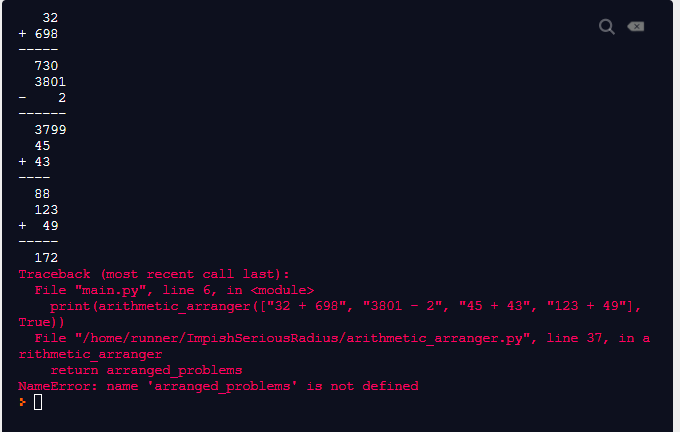# Arithmetic formatter: how to align output horizontally

Hi,
In arithmetic formatter I am able to print answers in a correct format I guess, but I wonder how can I align output horizontally as it shoud be according to requirements. I know that I need to use arranged problems, but I confused with how to use it.

this is my current progressmy code

``````def arithmetic_arranger(problems, bool):
for i in range(len(problems)):
space1 = " "
space2 = " "
dashes = "-"
digit1 = problems[i].split(" ")
digit2 = problems[i].split(" ")
sign = problems[i].split(" ")
if len(digit1) > len(digit2):
space1 = " " * (len(digit1) - len(digit2)) + " "
dashes = dashes * len(digit1) + "--"
if sign == "+":
elif sign == "-":
if len(digit1) < len(digit2):
space2 = " " * (len(digit2) - len(digit1)) + " "
dashes = dashes * len(digit2) + "--"
if sign == "+":
elif sign == "-":
#print(len(digit2), len(digit1))
if len(digit1) == len(digit2):
dashes = dashes * len(digit1) + "--"
if sign == "+":
elif sign == "-":